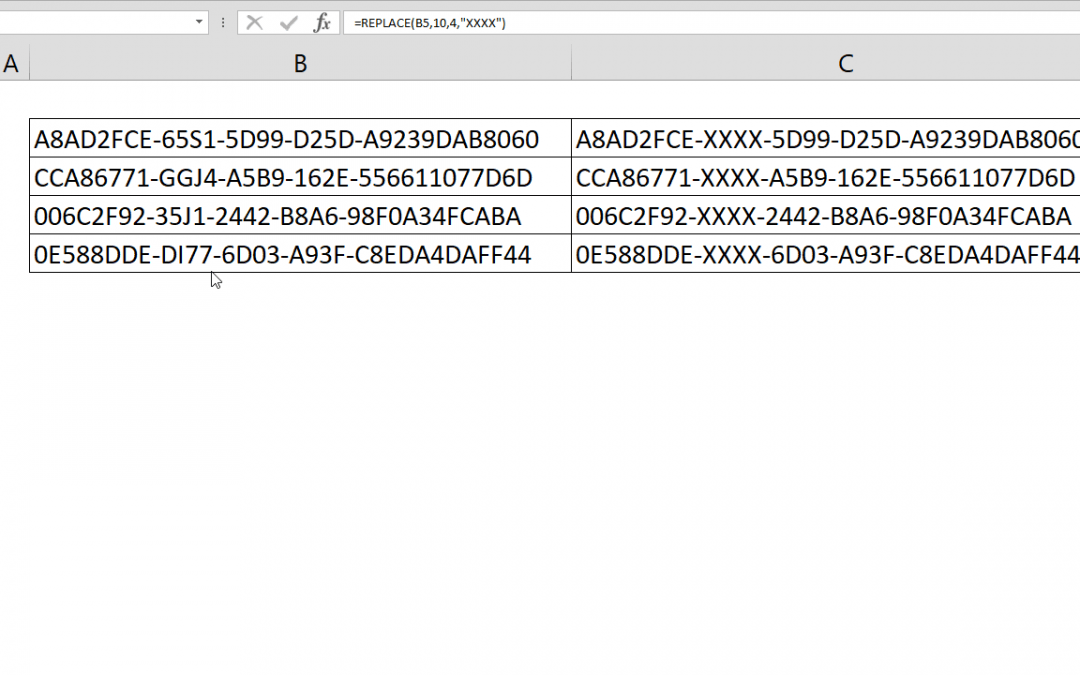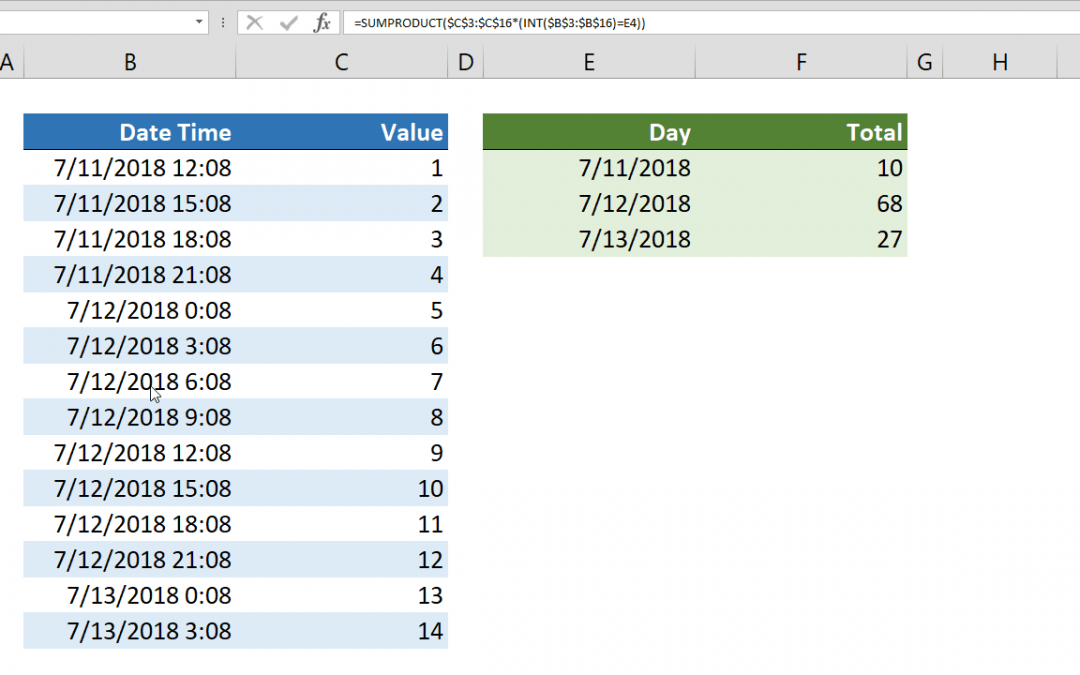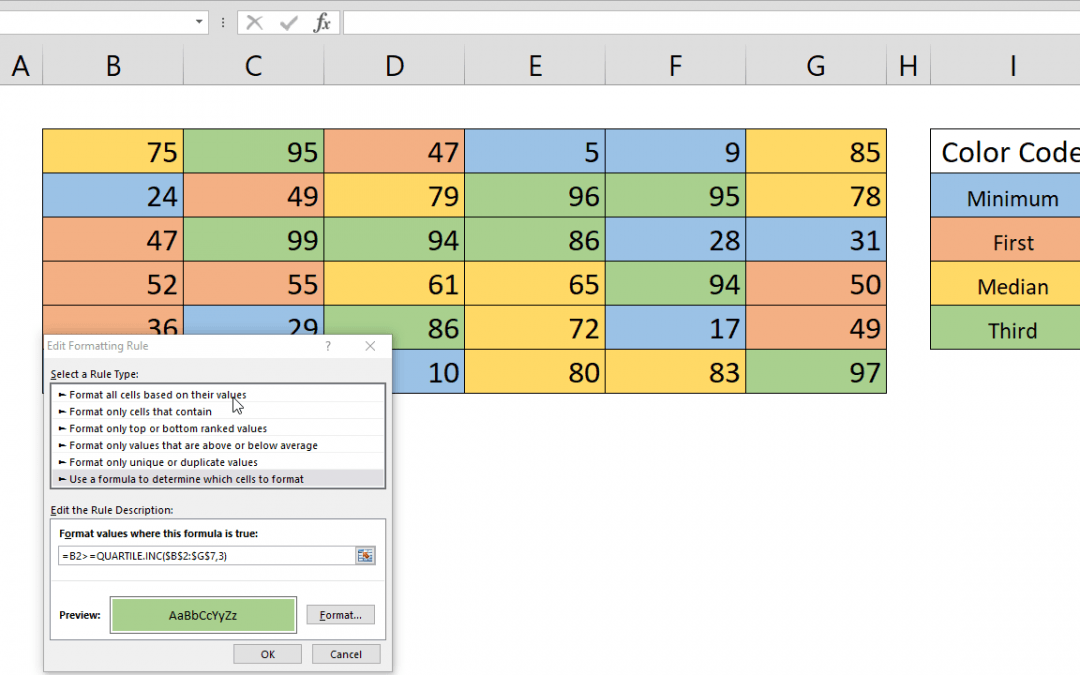## How to replace character in Excel by position

This article shows how to replace character in Excel by position using REPLACE function. If you are looking to replace a specific character please refer to article How to replace a character. Syntax =REPLACE( text, start position of replacement, number of characters...## Grouping Dates in Pivot Table

Excel already has functions like the SUMIF and the SUMIFS for summing data by groups. However, they won’t work when have date-time values combined. In this article we will cover grouping dates in Pivot Table. Steps Select a cell in your data Click Pivot Table...## How to sum by date in Excel without time

Excel already has functions like the SUMIF and the SUMIFS for summing data by groups. However; they can't work if you have date-time values combined. This article shows How to sum by date in Excel without time using SUMPRODUCT and INT functions. Syntax =SUMPRODUCT(...## How to calculate running totals in Excel Tables

This article shows how to calculate running totals in Excel Tables by using structured references. If your data is not in a Table, please continue with this article: How to calculate running count Syntax =SUM(INDEX([column of values],1):[@ column of values]) Steps...## How to highlight quartiles in Excel

It is often a good idea to highlight duplicate values in a data set to help easily identify the outliers. In this article we are going to explain how to find quartiles in Excel and highlight them dynamically with Conditional Formatting. Syntax = relative reference of...## How to create a Quarter formula in Excel

There is no specific Quarter formula in Excel. But an Excel quarter formula can be constructed by combining ROUNDUP and MONTH functions with custom number formatting. Syntax =ROUNDUP( MONTH( date value ) /3, 0 ) Steps Start with =ROUNDUP( function Continue with MONTH(...## How to generate Excel month names

This article shows you how to generate month list by formulas and how to display Excel month names instead of actual date values. We will use DATE, YEAR and MONTH formulas as well as Number Formatting. Syntax =DATE( YEAR( previous date ), MONTH( previous date )+1, 1)...## How to calculate Excel Table totals

This article shows how to calculate Excel table totals by using Excel Table which is one of the most underrated features in Excel. Steps Select a cell inside your table or whole range Press Ctrl + T or click Table icon under INSERT tab on the ribbon Make sure My table...